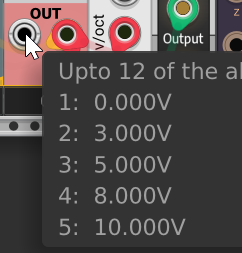# Confused by the polyoutput

I got stuck. Please pay attention to the .setVoltage() expression.

Following this code I get the expected result:

``````int cc=0;	// channel counter
outputs[POLY_ALLOWED_OUTPUT].channels=16;
for (int p=0;p<12;p++) {
if (paramVal[NOTE_PARAM+p]==1) {
outputs[POLY_ALLOWED_OUTPUT].setVoltage(p,cc);
cc++;
}
}
outputs[POLY_ALLOWED_OUTPUT].channels=cc;
``````Following this code I get the result I thought:

``````int cc=0;	// channel counter
outputs[POLY_ALLOWED_OUTPUT].channels=16;
for (int p=0;p<12;p++) {
if (paramVal[NOTE_PARAM+p]==1) {
outputs[POLY_ALLOWED_OUTPUT].setVoltage(p+0.12,cc);
cc++;
}
}
outputs[POLY_ALLOWED_OUTPUT].channels=cc;
``````

##But I’m clueless what’s going on here:

``````int cc=0;	// channel counter
outputs[POLY_ALLOWED_OUTPUT].channels=16;
for (int p=0;p<12;p++) {
if (paramVal[NOTE_PARAM+p]==1) {
outputs[POLY_ALLOWED_OUTPUT].setVoltage(p/12,cc);
cc++;
}
}
outputs[POLY_ALLOWED_OUTPUT].channels=cc;
``````But why? What’s the catch? I just don’t get it. I’d expect to have this result:

``````1:	0.000
2:	0.250
3:	0.417
4:	0.667
5:	0.833
``````

The compiler is doing integer division instead of floating point devision.

This is because both the numerator and denominator of the devision are integer values. Specifically `p` is an int and `12` is an integer literal.

There are several fixes. Simplest would be to change `12` to `12.0f`. This will work because as long at least one of the two operands used is a floating point value, the compiler will do floating point devision.

3 Likes

Also I noticed in your code you are directly setting channels here

``````outputs[POLY_ALLOWED_OUTPUT].channels=16;
``````

According to the comments in the source code, this is deprecated and an unstable part of the API. I.E. this code may break in the future. You should instead do this:

``````outputs[POLY_ALLOWED_OUTPUT].setChannels(16);
``````
2 LikesMany thanks, @Patheros!

I’m too embarrassed to tell you the amount of time I spent with debugging…

1 Like

You are welcome. I’m glad I could help. I’ve definitely spent my fair share of time tracking down bugs of all shapes and sizes2 Likes